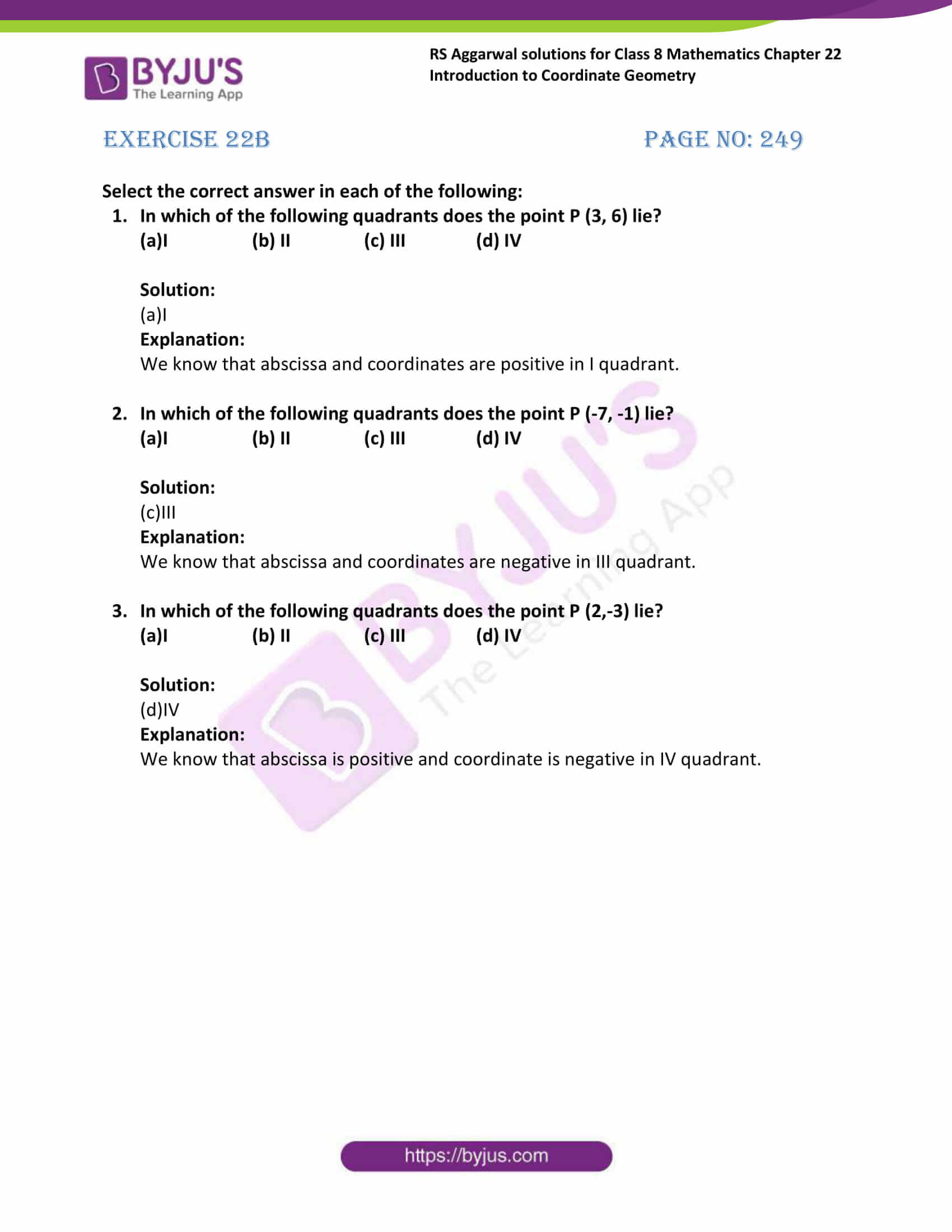# RS Aggarwal Solutions for Class 8 Maths Chapter 22 - Introduction to Coordinate Geometry Exercise 22B

RS Aggarwal Solutions for the Exercise 22B of Class 8 Maths Chapter 22, Introduction to Coordinate Geometry are available here. BYJU’S subject expert has uniquely solved the exercise for your better understanding. As this is the last exercise, it includes multiple-choice questions which enhance time management skills among the students. By practising the RS Aggarwal Solutions for Class 8, students will be able to grasp the concepts correctly.

## Download PDF of RS Aggarwal Solutions for Class 8 Maths Chapter 22 – Introduction to Coordinate Geometry – Exercise 22B### Access answers to Maths RS Aggarwal Solutions for Class 8 Chapter 22 – Introduction to Coordinate Geometry Exercise 22B

Exercise 22B Page No: 249

Select the correct answer in each of the following:

1. In which of the following quadrants does the point P (3, 6) lie?

(a)I (b) II (c) III (d) IV

Solution:

(a)I

Explanation:

We know that abscissa and coordinates are positive in I quadrant.

2. In which of the following quadrants does the point P (-7, -1) lie?

(a)I (b) II (c) III (d) IV

Solution:

(c) III

Explanation:

We know that abscissa and coordinates are negative in III quadrant.

3. In which of the following quadrants does the point P (2,-3) lie?

(a)I (b) II (c) III (d) IV

Solution:

(d)IV

Explanation:

We know that abscissa is positive and coordinate is negative in IV quadrant.

### Access another exercise of RS Aggarwal Solutions for Class 8 Maths Chapter 22 – Introduction to Coordinate Geometry

Exercise 22A Solutions 13 questions

## RS Aggarwal Solutions for Class 8 Maths Chapter 22 – Introduction to Coordinate Geometry Exercise 22B

Exercise 22B of RS Aggarwal Solutions for Chapter 22, Introduction to Coordinate Geometry deals with the basic concepts related to a cartesian plane and coordinate geometry. Some of the topics covered before this exercise are listed below.

• Definition of the cartesian plane
• Ordered pair

RS Aggarwal Solutions can help the students in exercising and learning each and every concept as it provides solutions to all questions asked in the RS Aggarwal textbook. Those who aim to score high in the Maths of Class 8 are advised to practice all the problems present in RS Aggarwal as many times as possible.